# ISRO Scientist or Engineer Mechanical 2013

Instructions

For the following questions answer them individually

Question 1

# A concentrated load is applied at the end of a cantilever as shown in Fig. The cross section of the beam is a square of side ‘a’ with a hole of dia ‘$$\frac{a}{2}$$’. The deflection at the tip of the cantilever is given by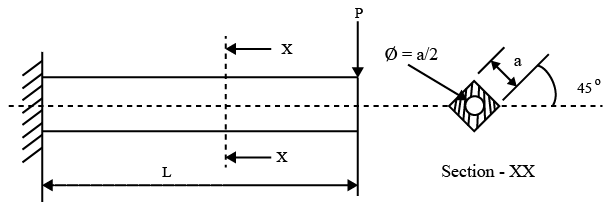Question 2

# A cantilever beam is subjected to a UDL. The cross section of the beam is a H-Section placed as shownin Fig. The bendingstress distribution across the cross section will be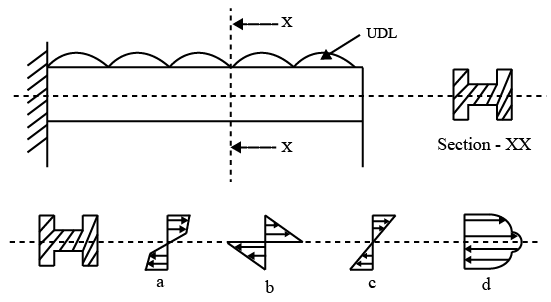Question 3

# A thick cylinder of inner dia ‘D’, wall thickness $$t_2$$ and length ‘L’ is sealed at its both ends with caps. The thickness of the cap is $$t_1$$. Allowable tensile yield stress = $$\sigma_y$$ and allowable shear stress = $$\tau_y$$. A gas is pumped into this cylinder at pressure ‘p’. The cap will yield in shear at circumference of diameter ‘D’ when the gas pressure applied is more than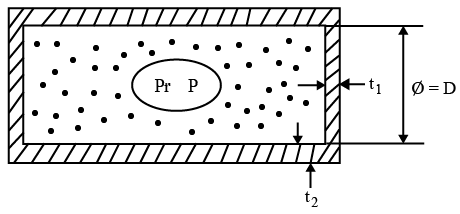Question 4

# An ‘SS’ tube is inserted into an ‘Al' tube.They are permently fixed at one end .The other end is attached to a rigid plate .A torque "T" is applied to the rigid plate .A torque "T" is applied to the rigid plate .The circumference of the 'Al' tube at dia 'D' at the plate end with respect to the fixed end rotates by a distance ....mm due to the TORQUE 'T'.The polar MOI & rigidity modulus of Al & ss are $$J_{Al},G_{Al}$$ and $$J_{ss},G_{ss}$$ respectively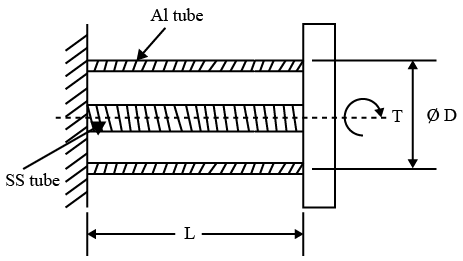Question 5

# A rod of 20 dia is fixed to the ceiling of a roof on one end.: A rotor of 50 kg mass is attached to the free end with bearings. The CG of the rotor is 10 mm away from the shaft axis. The rotor is rotating at 600 rpm. The max tensile stress (in N/sq.mm)in the rod is nearly equal to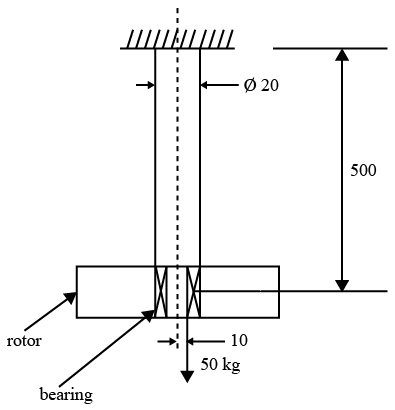Question 6

# An automotive engine having a mass of 135 kg is supported on springs with linear characteristics. Each of the 2 front springs have stiffness of 3 $$\frac{MN}{m}$$ while the stiffness of each of 2 rear springs is 4.5 $$\frac{MN}{m}$$ The engine speed (rpm) at which resonanceis likely to occur isQuestion 7

# A weighing $$\frac{m}{c}$$ consists of a 2 kg pan resting on a spring having linear characteristics. In this condition of resting on the spring, the length of spring is 200mm. Whena20 kg massis placed _on the pan,the length of the spring becomes 100mm. The undeformed length Lin mm and the spring stiffness K in $$\frac{N}{m}$$areQuestion 8

# A circular shaft is subjected to a torque ‘T’ and a Bending Moment M. Theratio of max. shear stress to max. bendingstressisQuestion 9

# A solid block ‘A’ wéighing ‘Q’ kg is resting on flat floor. A smooth cylinder ‘B’ weighing ‘P’ kg. is placed betweenthe solid A and the vertical wall as shownin fig. The friction between the cylinder, wail and the block A is negligible. The co-efficient of friction between the block A and floor is $$\mu$$ The minimum weight P required to disturb the block A is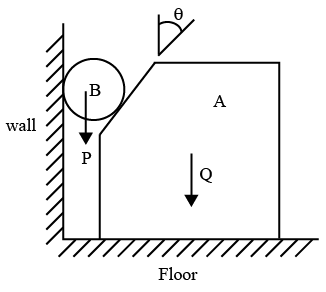Question 10

# A hydraulic jack is used to compress a spring as shown in the figure.Stiffness of spring is $$10^{5}$$ N/m By applying a pressure ‘p’ in the hydraulic cylinder, the spring gets compressed by 10 mm. The cross sectional area of the piston is 25 $$cm^{2}$$. The applied pressure‘p is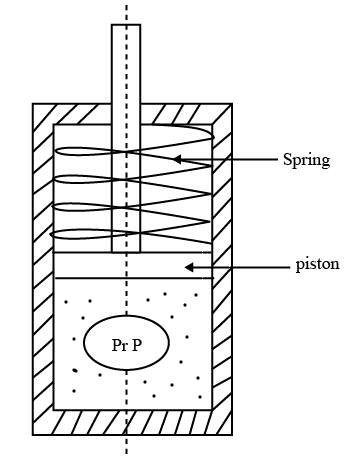OR